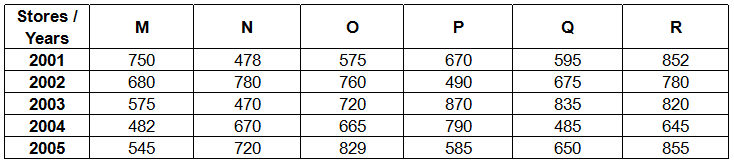## RBI Assistant Quant Test 13

Instructions

Read the following table carefully and answer the questions given below it.
Sales of Aviator sun-glasses by six stores M, N, O, P, Q, and R during 5 yearsQ 1

What is the respective ratio between the total number of sun glasses sold by store M in the years 2001, 2002 and 2003 and that sold by store P in the same years ?

Q 2

By what per cent is the number of sun glasses sold by store N in the year 2005 less than that sold by store R in the year 2003 ?

Q 3

What is the respective ratio between the average number of sun glasses sold by store P and store Q during all the given years ?

Q 4

By what per cent approximately is the total number of sun glasses sold by store P in the years 2003, 2004 and 2005 more than that sold by store in the same years ?

Q 5

What is the average number of sun glasses sold by store R in the given years ?